Home | Footings And Rafts

# Footings And RaftsTypes of foundation - Contact pressure distribution below footings and raft - Isolated and combined footings - Types and proportioning - Mat foundation- Types, applications uses and proportioning-- floating foundation.

FOOTINGS AND RAFTS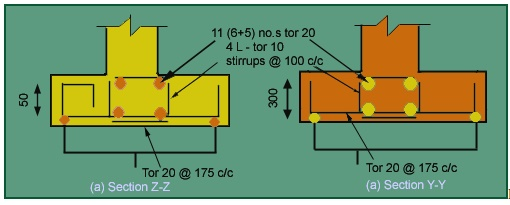P1=800kN

P2 =1000kN

qo =20 t/m2,M15,  fy=415kN/m2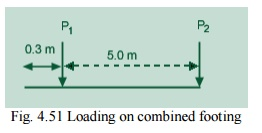Column size: 400x400mm.

See Fig 4.54 for details of footing.  Column design

Let pt=0.8%

Ax =.008A;  Ax=0.992A

Clause.39.3 of IS 456-2000

A=146763.8mm2

Ax =1174.11 mm2,  =145589.746mm2

Provide footing of 400x400size for both columns.

Using 8-16 j as main reinforcement and 8   j  @250c/c as lateral tie

Design of FootingFig. 4.52 Forces acting on the footing

R =1800 kN acting 3.08m from the boundary.

Area of the footing :

Taking length L=6m, Depth of footing  Df=0.9m,Width of footing,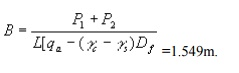=1.549m.

Therefore, provide footing of dimension 6m x 1.6m

Soil Pressure q = 180/ 6 x 1.6 =18.75 t/m2< 20 t/m2 OK.

qu=28.125 t/m2

Soil pressure intensity acting along the length =B x  =1.6x28.125 =45t/m.

RB =119.88kN, RC =150.12kN.

Thickness of Footing

i. Wide beam shear:

Maximum shear force is on footing C,SF=115.02KN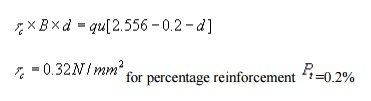for percentage reinforcement Pt =0.2%

0.32 x d x 1.6=45 [2.556-0.2-d]

d=1.1mfor percentage reinforcement  =0.6% 0.6 x d x 1.6=45 [2.556-0.2-d] d=0.847m.D=900mm.OK.ii.Two way Shear Thickness of Footing

i. Wide beam shear:

Maximum shear force is on footing C,SF=115.02KNfor percentage reinforcement  Pt=0.2%

0.32 x d x 1.6=45 [2.556-0.2-d]

d=1.1m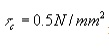for percentage reinforcement Pt =0.6%

0.6 x d x 1.6=45 [2.556-0.2-d]

d=0.847m.D=900mm.OK.ii.Two way Shear

Column B

d=0.415m.

Column A

2d[(0.4+d)+(0.42+d/2)] x 96.8=120-28.125[(0.4+d)(0.42+d/2)] d=0.3906m

dreqd =0.85mm

Dprovided =900mm, dreqd =850mm.OK.

Flexural reinforcement

Along Length Direction

Mu/bd2=1.15N/mm2

Table 1of SP16

Pt  =0.354%

Ptprovided=0.6%

Astrequired=5100 mm2/mm

Provide 28 j @120mmc/c at top and bottom of the footing

Along width directionRaft Footing Design the raft footing for the given loads on the columns and spacing between the columns as shown below.Fig 4.57 column locations and intensity of loads acting on the raft

a) Column sizes

Take size of the columns are as: 300*450 mm for load of less than 115 ton

450*450 mm for a load of greater than 115 ton

Thickness of raftTwo way shear

The shear should be checked for every column, but in this case because of symmetry property checking for 115 t, 150 t, and 55 t is enough.

For 150 t columnFig 4.58 section for two way shear for 150 t column

IS: 456-1978,

bc=450/450=1.04(0.45+d)*d*96.8=150*1.5-5.607(0.45+d)2

Therefore d=0.562 m

For 115 t column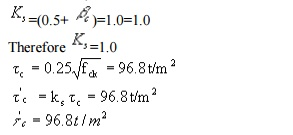Fig 4.59 section for two way shear for 115 t column

2(0.45+d+0.15+0.3+d/2) d*96.8=115*1.5-5.607(0.45+d)(0.3+0.15+0.5d)

Therefore d=0.519 m

For 55 t columnFig 4.60 section for two way shear for 55 t column

2(0.45+0.075+0.5d+0.15+0.3+0.5d) d*96.8=55*1.5-5.607(0.45+0.5d+0.075)(0.3+0.5d+0.15)

Therefore d=0.32 m

The guiding thickness is 0.562m and code says that the minimum thickness should not be less than 1.0m.

let provide a overall depth of 1.1m=D

dpdv =1100-75-20/2=1015mm.

To calculate k &  l Stiffness factors

There are two criterions for checking the rigidity of the footing:

Plate size used is 300*300 mm.

For clays:  Mus=0.5,If column spacing is less than 1.75/ l, then the footing is said to be rigid.

Therefore the given footing is rigid.

One criterion showing the footing is flexible and another showing that the given footing is rigid. Both are contradicting each other, so design the footing for both criterions.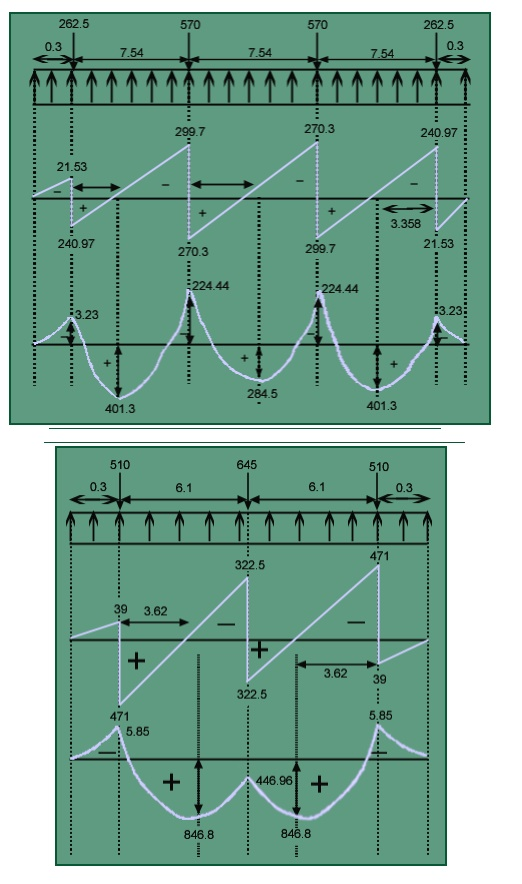Reinforcement in width directionFrom SP-16 graphs

Pt =0.102%, but minimum is 0.12%.

Provide 20 mm diameter bars @250 c/c along shorter direction in bottom.

Reinforcement in length directionProvide 20 mm diameter bars @250 c/c in longer direction.

Clause 33.3.1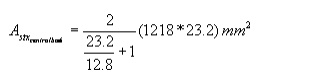Provide 20 mm diameter bars @ 200 c/c in central band and 20 mm diameter bars @300 c/c at other parts along shorter direction at bottom.

Shear (wide beam shear criterion)

In width direction=0.235 N/mm2 <  r(0.27 N/mm2)

Therefore no shear reinforcement is required.

Along the width directionFig. 4.63 Shear Force and Bending Moment Diagrams of strips 1 and 4

In width direction: Strip1/4:-  =141.2tm

Strip2/3Fig. 4.64 Shear Force and Bending Moment Diagrams of strips 2 and 3

Strip 2/3Mu =282.36tm

M/bd2 = 0.364N/mm2

Minimum  Pt=0.12%has to be provided.

Provide 20  j @200c/c in centre band and 20 j @300c/c at other parts along the shorter direction.

1. Shear check

Along width direction:-

For strip1/4:

Va=76.35tFor strip 2/3:

Va=159.14 tHence no shear reinforcement is required.

Development LengthAt the ends, length of bar provided=150mm.

Extra length to be provided=1128.3-150-8x20=818.3mm.

Provide a Development length of 850mm

3. Transfer of load at the base of the column:-

For end column;

A1=2650X2725=7.22125x106mm2

A2=300x450=135000mm2Study Material, Lecturing Notes, Assignment, Reference, Wiki description explanation, brief detail
Civil : Foundation Engineering : Footings And Rafts : Footings And Rafts |

Related Topics

Civil : Foundation Engineering : Footings And Rafts